# Pythagorean Theorem Quiz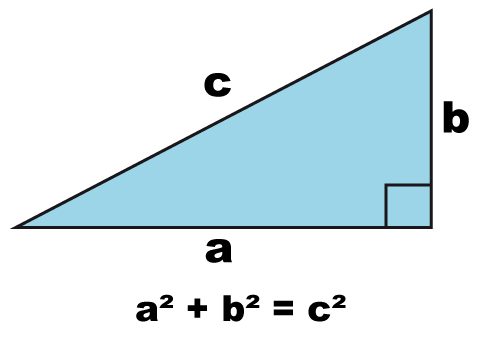We thoroughly check each answer to a question to provide you with the most correct answers. Found a mistake? Let us know about it through the REPORT button at the bottom of the page.

## Intro

Pythagorean Theorem Quiz1. Use the Pythagorean Theorem to see if the measurements below can form a right triangle. ***** a = 5 in. b = 12 in. c = 13 in.

• Yes, it is a right triangle.
• No, it is not a right triangle
• There is not enough info.

2. a = 6.4, b = 12, c = 12.2 is this a right triangle?

• yes
• no

3. a = 2.1, b = 7.2, c = 7.5 is this a right triangle?

• yes
• no

4. If a triangle has a side a=5 and b=12, what is c?

• 10
• 17
• 169
• 13

5. Which three side lengths form a right triangle?

• 5, 12, 13
• 4, 5, 7
• 3, 6, 9
• 1, 3, square root of 11

6. Use the Pythagorean Theorem to see if the measurements below can form a right triangle. **** a= 6 cm, b= 8 cm, c = 10 cm

• Yes, it is a right triangle.
• No, it is not a right triangle
• There is not enough info.

7. What is the length of x?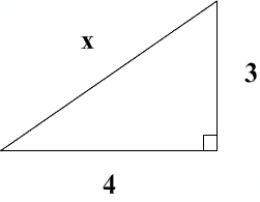• 5
• 7
• 8
• 25

8. Solve for c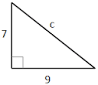• 33
• 11.2
• 11.4
• 13

9. Solve for x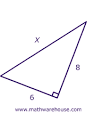• 10
• 11.4
• 13
• 2

10. Find the hypotenuse in the image shown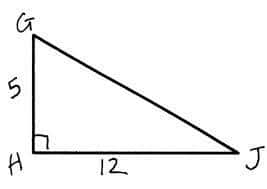• 169
• 13
• 14.4
• 17

11. Do the segment lengths 9, 12, and 15 form a right triangle?

• Yes
• No
• Maybe
• Pythagoras

12. Do the segment lengths 9, 11 and 15 cm form a right triangle?

• yes
• no
• maybe
• pythagoras

13. Given the 3 lengths of a triangle are 6 in, 8 in and 10 in.  Will this make a right triangle?

• yes
• no
• maybe
• 22

14. The Pythagorean Theorem ONLY works on which triangle?

• obtuse
• scalene
• isosceles
• right

15. What is the name of the longest side of a right-angled triangle?

• Pythagoras
• Hypotenoose
• Hipotenuse
• Hypotenuse

16. What kind of triangles does the Pythagorean Theorem work with?

• Right
• Left
• Isosceles
• Equilateral

17. What is the formula for Pythagoras theorem if in a right angles triangle a and b are the short sides and c is the longest side?

• a2 x b2 = c2
• a + b = c
• a2 + a2 = c2
• a2 + b2 = c2

18. Does an 8, 15, 16 triangle have a Right Angle?

• yes
• no

19. To get from point A to point B you must avoid walking through a pond.  To avoid the pond, you must walk 34 meters south and 41 meters east.  To the nearest meter, how many meters would be saved if it were possible to walk through the pond?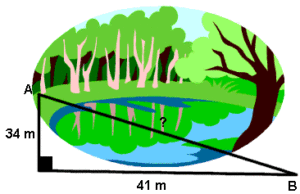• 35
• 22
• 58
• 75

20. John runs diagonally across a rectangular field that has a length of 40 yards and a width of 30 yards, as shown in the diagram.What is the length of the diagonal, in yards, that John runs?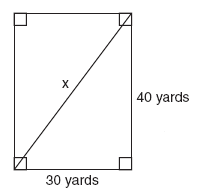• 50
• 60
• 70
• 80

21. Does this triangle have a Right Angle?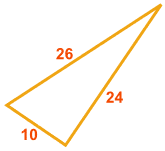• No
• Yes

## Terms and Answers to Learn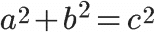Pythagorean Theorem Formula
Pythagorean Theorem Definition
A right triangle (with a 90 degree angle) is composed of two legs and a hypotenuse (side opposite the right angle).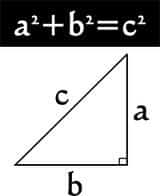Pythagorean TheoremPythagorean Theorem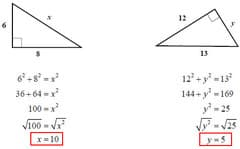Example: Pythagorean Theorem
Legs
Sides that are adjacent (same vertex, share a common side) the right angle. There are two.
Hypotenuse
The side opposite the right angle
Right Triangle
A triangle that has one right angle (90 degrees) with 2 legs, and one hypotenuse.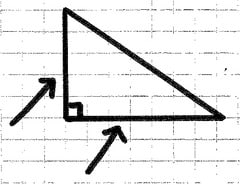leg
Either of the two shortest sides of a right triangle, they meet at a common vertex to form a right angle.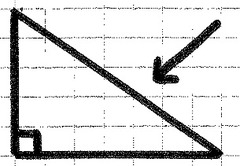hypotenuse
The longest side of a right triangle. It is always opposite of, and never is a part of, the right angle.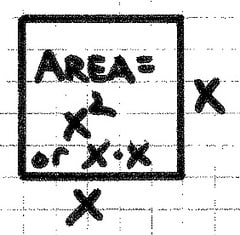square
The result of multiplying a number by itself.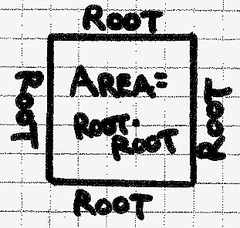root (of a square)
Any side of a square. A number which, when multiplied by itself, makes a square. One of the dimensions of a square.pythagorean theorem
The sum of the squares of the legs of a right triangle is equal to the square of the hypotenuse.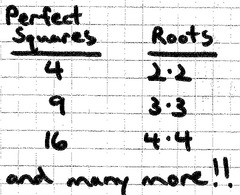perfect square
A square with a whole number root.Pythagoras
Greek philosopher, 570-495 BC. There is no evidence that Pythagoras himself worked on or proved the Pythagorean Theorem, which was used previously by Babylonians and Indians.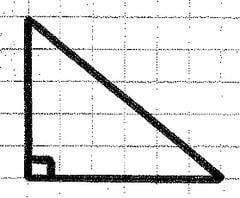right triangle
A triangle that contains a right angle.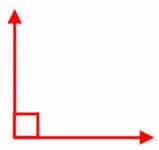right angle
An angle of exactly 90 degrees.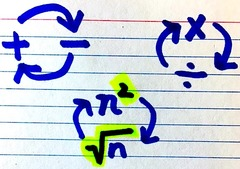inverse operations
Math operations that reverse the effect of each other.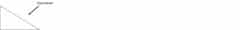c, and the longest side of a right triangle
Hypotenuse
labeled a and b, the other two sides of the right triangle
Leg
the square of the hypotenuse is equal to
the sum of the squares of the other two sides.
Pythagorean Theorem
5
a=3 b=4 c=?
25
a=7 b=24 c=?
10
a=6 b=8 c=?
No
Is this a right triangle: a=4, b=6, c=9
yes
Is this a right triangle: a=5 b=12 c=13
a triangle where one angle is guaranteed to be 90 degrees.
Right Triangle
yes
Would these three sides form a right angle 8, 15, 17
12
Which side length would be considered c? 9,12,10
12
Find b: a=5 b=? c=13Either of the two shortest sides of a right triangle, they meet at a common vertex to form a right angle.
legThe longest side of a right triangle. It is always opposite of, and never is a part of, the right angle.
hypotenuseThe result of multiplying a number by itself.
squareThe sum of the squares of the legs of a right triangle is equal to the square of the hypotenuse.
pythagorean theoremA square with a whole number root.
perfect squareGreek philosopher, 570-495 BC. There is no evidence that Pythagoras himself worked on or proved the Pythagorean Theorem, which was used previously by Babylonians and Indians.
PythagorasA triangle that contains a right angle.
right triangle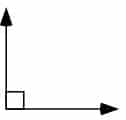An angle of exactly 90 degrees.
right angleMath operations that reverse the effect of each other.
inverse operations
A right triangle (with a 90 degree angle) is composed of two legs and a hypotenuse (side opposite the right angle).
Pythagorean Theorem Definition
Sides that are adjacent (same vertex, share a common side) the right angle. There are two.
Legs
The side opposite the right angle
Hypotenuse
A triangle that has one right angle (90 degrees) with 2 legs, and one hypotenuse.
Right Triangle
Right triangle geometric means formula
a=square root of b*c
d=square root of b+c * b
e=square root of b+c * c
How to recognize a right triangle
c2=a2+b2
How to recognize an obtuse triangle
c2>a2+b2
How to recognize an acute triangle
c2<a2+b2
How to solve 30-60-90 triangles
hypotenuse is 2b, legs are b and b square root of 3
How to solve 45-45-90 triangles
hypotenuse is a square root of 2, legs are same length